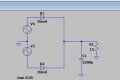help with a circuit (Rectifying circuit)

felastian123

Joined Oct 10, 2021
3
Hello, for the following circuit I am asked to calculate the average current through the diode, the capacitor and the load in permanent regime, in addition to finding the average current in one of the diodes with the values of its repetitive peaks, I would appreciate it if someone Can you give some advice or a book where you explain what I need, thank you very much.ericgibbs

Joined Jan 29, 2010
14,715
hi f123,
Welcome to AAC.
E

Attachments

• 788.3 KB Views: 9
Last edited:
•felastian123

MrAl

Joined Jun 17, 2014
8,617
Hello, for the following circuit I am asked to calculate the average current through the diode, the capacitor and the load in permanent regime, in addition to finding the average current in one of the diodes with the values of its repetitive peaks, I would appreciate it if someone Can you give some advice or a book where you explain what I need, thank you very much. View attachment 250703
Hello and welcome to the forum.

What do you mean by "permanent regime"? Could you state that in another way perhaps?

•felastian123

Sfucci

Joined Oct 21, 2021
1
That pdf you uploaded Is awesome ty

•felastian123

Papabravo

Joined Feb 24, 2006
17,617
Hello and welcome to the forum.

What do you mean by "permanent regime"? Could you state that in another way perhaps?
What is the amplitude, phase, and frequency of the sources V3 and V4?
You do realize that there are two (count 'em 2) diodes in your schematic.

•felastian123

MrChips

Joined Oct 2, 2009
25,053
•felastian123

felastian123

Joined Oct 10, 2021
3
Hello and welcome to the forum.

What do you mean by "permanent regime"? Could you state that in another way perhaps?
stationary state, ty

MrChips

Joined Oct 2, 2009
25,053
stationary state, ty
The expression we use is "steady state" o "estado estable" en español.

•felastian123

MrAl

Joined Jun 17, 2014
8,617
stationary state, ty
Thanks, and by that i assume you mean steady state as others have mentioned.
In that case, i presume that you assume that the capacitor has the average DC voltage across it at all times even when the diode(s) conduct, and that would be a typical strategy for solving a circuit like this.

So i can at least give a hint here although i dont know what you have done with circuits like this in the past. The hint is:
If by assumption the cap always has the same DC voltage across it at all times then it's efficiency is 100 percent meaning the only power dissipation occurs in the resistive load, hence any calculations will not involve the capacitor at all except as a constant voltage source. If there is any dissipation in the diodes that would depend on what model you are using for the diodes here and i am guessing either no voltage drop when conducting or some fixed voltage when conducting, but correct me if i am wrong.

The full blown analysis of these kinds of rectifier circuits is very interesting but very complicated as it involves the actual conduction times of the diodes which also has to be solved for so most people dont want to be bothered by that.

Last edited:

neonstrobe

Joined May 15, 2009
180
Are you able to assume that the diodes are ideal (no forward voltage drop when conducting and no leakage in the reverse state) and that there is no impedance in the voltage sources?
Does that help to get going with the answer?